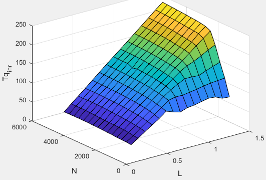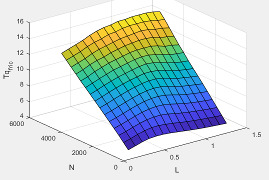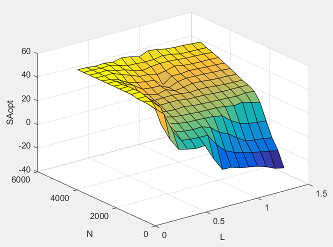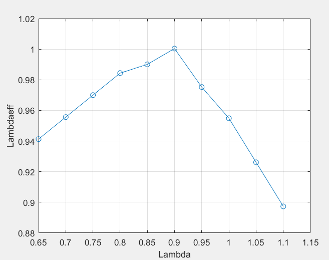## SI Engine Torque Structure Model

The spark-ignition (SI) engine implements a simplified version of the SI engine torque structure calculation used in a Bosch Engine Management System (EMS). For the torque structure estimation calculation, the block requires calibration tables for:

• Inner torque — Maximum torque potential of the engine at a given speed and load

• Friction torque — Torque losses due to friction

• Optimal spark — Spark advance for optimal inner torque

• Spark efficiency — Torque loss due to spark retard from optimal

• Lambda efficiency — Torque loss due to lambda change from optimal

• Pumping torque — Torque loss due to pumping

The tables available with Powertrain Blockset™ were developed with the Model-Based Calibration Toolbox™.

Lookup TableUsed to DeterminePlot

Inner torque, ${f}_{Tqinr}$

$T{q}_{inr}={f}_{Tqinr}\left(L,N\right)$

The inner torque lookup table, ${f}_{Tqinr}$, is a function of engine speed and engine load, $T{q}_{inr}={f}_{Tqinr}\left(L,N\right)$, where:

• $T{q}_{inr}$ is inner torque based on gross indicated mean effective pressure, in N·m.

• L is engine load at arbitrary cam phaser angles, corrected for final steady-state cam phaser angles, dimensionless.

• N is engine speed, in rpm.Friction torque, ${f}_{Tfric}$

${T}_{fric}={f}_{Tfric}\left(L,N\right)$

The friction torque lookup table, ${f}_{Tfric}$, is a function of engine speed and engine load, ${T}_{fric}={f}_{Tfric}\left(L,N\right)$, where:

• ${T}_{fric}$ is friction torque offset to inner torque, in N·m.

• L is engine load at arbitrary cam phaser angles, corrected for final steady-state cam phaser angles, dimensionless.

• N is engine speed, in rpm.Pumping torque, ƒTpump

`Tpump=ƒTpump(L,N)`

The pumping work lookup table, ƒTpump, is a function of engine load and engine speed, `Tpump=ƒTpump(L,N)`, where:

• Tpump is pumping work, in N·m.

• L is engine load, as a normalized cylinder air mass, dimensionless.

• N is engine speed, in rpm.Optimal spark, ${f}_{SAopt}$

$S{A}_{opt}={f}_{SAopt}\left(L,N\right)$

The optimal spark lookup table, ${f}_{SAopt}$, is a function of engine speed and engine load, $S{A}_{opt}={f}_{SAopt}\left(L,N\right)$, where:

• SAopt is optimal spark advance timing for maximum inner torque at stoichiometric air-fuel ratio (AFR), in deg.

• L is engine load at arbitrary cam phaser angles, corrected for final steady-state cam phaser angles, dimensionless.

• N is engine speed, in rpm.Spark efficiency, ${f}_{Msa}$

$\begin{array}{l}{M}_{sa}={f}_{Msa}\left(\Delta SA\right)\\ \Delta SA=S{A}_{opt}-SA\end{array}$

The spark efficiency lookup table, ${f}_{Msa}$, is a function of the spark retard from optimal

`$\begin{array}{l}{M}_{sa}={f}_{Msa}\left(\Delta SA\right)\\ \Delta SA=S{A}_{opt}-SA\end{array}$`

where:

• ${M}_{sa}$ is the spark retard efficiency multiplier, dimensionless.

• $\Delta SA$is the spark retard timing distance from optimal spark advance, in deg.Lambda efficiency, ${f}_{M\lambda }$

${M}_{\lambda }={f}_{M\lambda }\left(\lambda \right)$

The lambda efficiency lookup table, ${f}_{M\lambda }$, is a function of lambda, ${M}_{\lambda }={f}_{M\lambda }\left(\lambda \right)$, where:

• ${M}_{\lambda }$ is the lambda multiplier on inner torque to account for the air-fuel ratio (AFR) effect, dimensionless.

• $\lambda$ is lambda, AFR normalized to stoichiometric fuel AFR, dimensionless.The engine brake torque is a based on inner torque with lambda efficiency, spark retard efficiency multipliers, pumping torque, and a friction torque offset

`${T}_{brake}={M}_{\lambda }{M}_{sa}T{q}_{inr}-{T}_{fric}-{T}_{pump}$`

To account for thermal effects, the torque structure model corrects the friction torque calculation as a function of coolant temperature.

`$\begin{array}{l}{T}_{fric}={M}_{fric}{f}_{Tfric}\left(L,N\right)\\ {M}_{fric}={f}_{fric,temp}\left({T}_{coolant}\right)\end{array}$`

The pumping torque is a function of engine speed and engine speed.

`${T}_{pump}={f}_{Tpump}\left(L,N\right)$`

 $S{A}_{opt}$ Optimal spark advance timing for maximum inner torque at stoichiometric air-fuel ratio (AFR) $\Delta SA$ Spark retard timing distance from optimal spark advance $SA$ Spark advance timing $L$ Engine load at arbitrary cam phaser angles, corrected for final steady-state cam phaser angles N Engine speed ${M}_{\lambda }$ Lambda multiplier on inner torque to account for the AFR effect $\lambda$ Lambda, AFR normalized to stoichiometric fuel AFR ${M}_{sa}$ Spark retard efficiency multiplier ${f}_{Msa}$ Spark efficiency lookup table to account for torque loss due to spark retard from optimal ${f}_{Tfric}$ Friction torque lookup table to account for torque losses due to friction ${f}_{M\lambda }$ Lambda efficiency lookup table to account for torque loss due to lambda change from optimal ${f}_{SAopt}$ Optimal spark lookup table, for maximum inner torque as a function of engine speed and load ${f}_{Tqinr}$ Inner torque lookup table, for maximum torque potential of the engine at a given speed and load ${T}_{brake}$ Engine brake torque after accounting for spark advance, AFR, and friction effects ${T}_{fric}$ Friction torque offset to inner torque $T{q}_{inr}$ Inner torque based on gross indicated mean effective pressure Tpump Pumping torque Mfric Friction torque modifier Tcoolant Coolant temperature

 Gerhardt, J., Hönninger, H., and Bischof, H., A New Approach to Functional and Software Structure for Engine Management Systems – BOSCH ME7. SAE Technical Paper 980801, 1998.

## SupportGet trial now